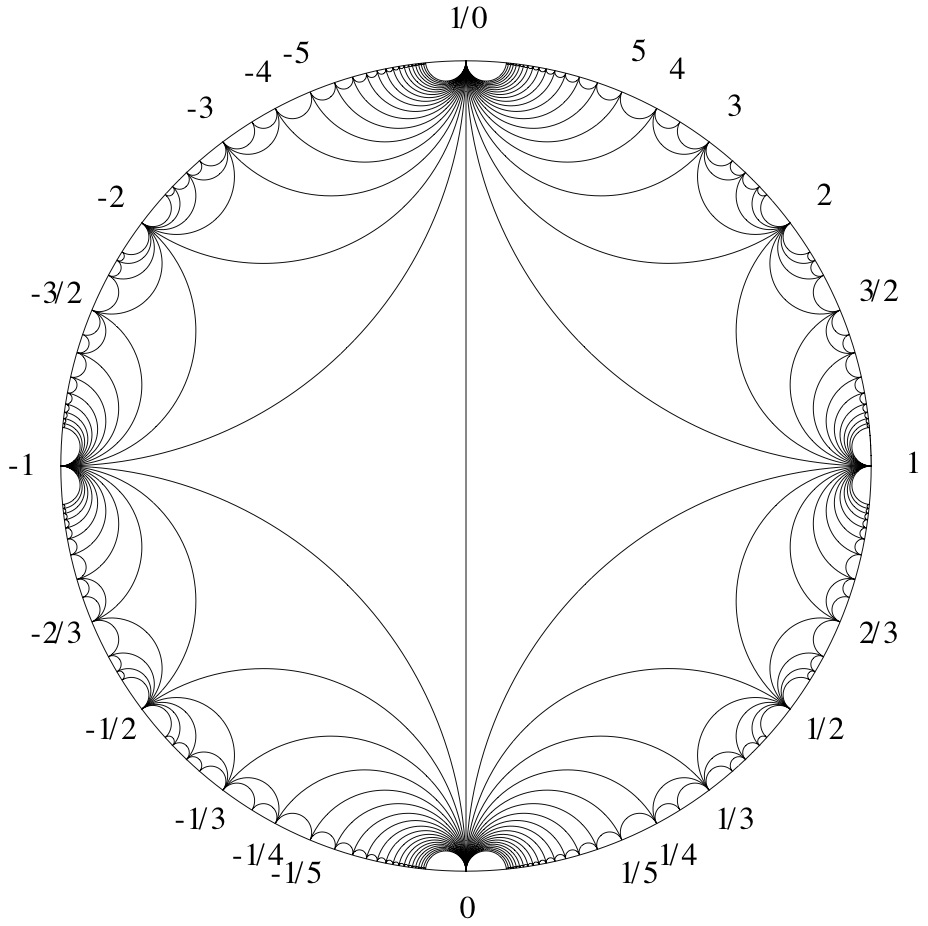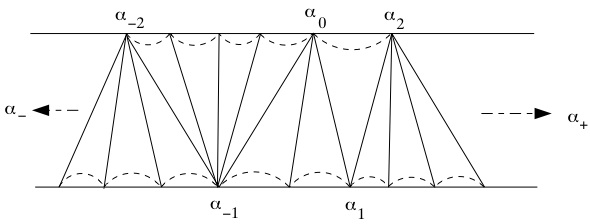# Is there an elementary proof of a result about the parity of the period of the repeating block in the continued fraction expansion of square roots

It is a known fact that for a Prime $P$, $P\equiv 1$ mod $4$ iff the length of the period in the repeating block for the continued fraction expansion of $\sqrt{P}$ is odd. I have an elementary proof of this using the classical result: $P\equiv 1$ mod $4$ iff $x^2-Py^2=-1$ has integer solutions and a proof that $x^2-Py^2=-1$ has integer solutions iff the length of the period in the repeating block for the continued fraction expansion of $\sqrt{P}$ is odd.

I have tried repeatedly to give a direct elementary proof that $P\equiv 1$ mod $4$ implies that the length of the period in the repeating block for the continued fraction expansion of $\sqrt{P}$ is odd, but cant seem to figure it out. (I have a direct elementary proof of the converse)

Does anyone know of an elementary proof of this result or where I may find one?

• I'd be curious to see the elementary proof that P prime, P = 1 mod 4 implies integer solutions to x^2 - P y^2 = -1. (I'm afraid I don't know the answer to your question.) – David E Speyer Jan 29 '10 at 2:31
• David, here is a sketch: Let x + ysqrt(p) > 1 be the least unit greater than 1 with norm 1. Then x and y have to be positive integers (any unit in Z[sqrt(d)] which is greater than 1 must have positive coeff. wrt the basis {1,sqrt(d)}). Show x is odd, y is even and from the equation py^2 = (x+1)(x-1) get an equation m^2 - pn^2 = 1 where 1 < m < x. This can't go on forever, so some unit in Z[sqrt(p)] must have norm -1. – KConrad Jan 29 '10 at 4:20
• An Elementary proof of the converse: Let r denote the length of the repeating block in the Continued fraction (CF) for sqrt(P), P Prime. If r is odd, then by Lagrange sqrt(P)=[a0,a1,a2,...,am,am,...a2,a1,2a0], where the integers a1,a2,...,am,am,...a2,a1,2a0 are repeating. Then the (m+1)st complete quotient, say A=[am,...,a1,2a0,a1,...,am], which is purely periodic. But then A=B, where B denotes the CF for A but with the period reversed. Thus, by Galois' Theorem, AA'=BA'=-1 and A is a reduced Quad. irrational; hence A=(a+sqrt(P))/b, for a,b in Z. So, AA'=-1 <=> P=a^2+b^2. – Jason Smith Jan 29 '10 at 5:24
• Nice proof KConrad. If anyone is interested I have a proof of this using Continued Fractions. – Jason Smith Jan 29 '10 at 5:30
• Jason: I would like very much to see the proof using continued fractions that you refer to. – Allen Hatcher Jan 30 '10 at 20:36

This doesn't answer your question, but it might gives some intuition as to why this is true. Given the fact that there exists a solution to the equation $x^2-Py^2=-1$ when $P\equiv 1(\mod 4)$, one sees that the matrix $$\begin{pmatrix}x & Py \\\ y & x\end{pmatrix}$$ fixes $\pm\sqrt{P}$ (under the action of $PGL_2(\mathbb{Z})$ on $\mathbb{RP}^1=\partial_{\infty} \mathbb{H}^2$). The conjugacy class of a primitive matrix in $GL_2(\mathbb{Z})$ (which is not reducible or finite order) is determined by the closed geodesic on the modular orbifold that it represents. This in turn is determined by a sequence of triangles which the geodesic crosses in the Farey graph:These triangles come in bunches sharing a common vertex, where the number in each bunch corresponds to coefficients of the continued fraction expansion. The matrix is conjugate to $$\pm \left[\begin{array}{cc}1 & a_1 \\\ 0 & 1\end{array}\right] \left[\begin{array}{cc}1 & 0 \\\ a_2 & 1\end{array}\right] \cdots \left[\begin{array}{cc}1 & a_{2n} \\\ 0 & 1\end{array}\right]$$ if the determinant is 1, and to $$\pm \left[\begin{array}{cc}1 & a_1 \\\ 0 & 1\end{array}\right] \left[\begin{array}{cc}1 & 0 \\\ a_2 & 1\end{array}\right] \cdots \left[\begin{array}{cc}1 & 0 \\\ a_{2n-1} & 1\end{array}\right] \left[\begin{array}{cc}0 & 1 \\\ 1 & 0\end{array}\right]$$ if the determinant is $-1$.[Remark: the labels in this figure don't quite correspond to the matrices - it should be $a_i$'s instead of $\alpha_i$'s, and $\alpha_{\pm}$ should be $\pm\sqrt{P}$]

The number of such factors corresponds precisely to the period of the continued fraction expansion of fixed points of the matrix, since the closed geodesic is asymptotic in $\mathbb{H}^2$ to the geodesic connecting $\infty$ to $\sqrt{P}$, whose Farey sequence gives rise to the continued fraction expansion of $\sqrt{P}$. This number is even if and only if the matrix is orientation preserving, which is if and only if the determinant is 1. So the determinant is $1$ if and only if the continued fraction has even period, and the determinant is $-1$ if and only if the continued fraction has odd period, corresponding to $P\equiv 1(\mod 4)$.

• There is a nice dual picture using Ford circles. I think if you draw a vertical line from $\pm\sqrt{p}$ to $i\infty$, the Ford circles that it intersects are tangent to the real line at the convergents. – S. Carnahan Jan 30 '10 at 5:43
• Nice pictures! I taught an undergraduate number theory course from this point of view (The Farey diagram) last semester, the notes for which are available here: math.cornell.edu/~hatcher/TN/TNpage.html See Chapters 1 and 2 in particular. Conway's topographs also form an integral part of the story. (My apologies for the shameless self-promotion!) When I revise the notes I'll have to add the nice fact discussed in the original post above, which was new to me. Thanks to all for the great answers! – Allen Hatcher Jan 30 '10 at 5:49
• Thank you Agol! My proof as stated in the question uses only what I learned from reading C.D. Olds' 'Continued Fractions' and Edward J. Barbeau's 'Pell's Equation' and a lot of thinking. I have virtually no exposure to Faray Diagrams but have a good foundation in modern algebra. Is there any books at the master's level that you would suggest so that I can understand your answer? – Jason Smith Jan 30 '10 at 19:04
• Oh, Allen Hatcher has some nice info on this. Thanks Allen. – Jason Smith Jan 30 '10 at 19:43
• @joro: I think the question is for square roots of primes. – Ian Agol Oct 8 '11 at 19:11

See Nagell, Introduction to number theory, Theorem 107. Originally it was proved by Legendre, see Dickson, L. E. History of the theory of numbers. Vol. II, p. 365. There are more examples due to Dirichlet, see Dirichlet's Werke, Bd. 1 (1889), pp. 219-236.

An extended bibliography on negative Pell’s equation can be found in Gerasim, I.-Kh.I. On the genesis of Redei’s theory of the equation x 2 -Dy 2 =-1. (Russian) Zbl 0731.01014 Istor.-Mat. Issled. 32/33, 199-211 (1990) (available in electronic form).